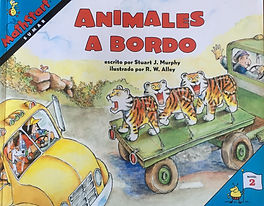Back to Children's LiteratureAnno's Counting Book##### by Stuart J. Murphy
###### Eleven truck drivers deliver thirty-nine animals and the top of a merry-go-round ride. Add up the tigers, swans, frogs, horses, and pandas as they pass by.

Resources for Animals on Board:

Full Book Review (English)

Full Book Review (Spanish)

Brief Book Review (English)

Brief Book Review (Spanish)

Where are all the animals going?

Activities To Do Together:

• Talk about how the numbers, addition signs, and equal signs represent what is happening in the pictures of this story.

• Talk about what it means to be equal.

• Make your own addition equations. For example, if you have three crayons and four pencils write an equation to show how many objects you have, 3 + 4 = 7.

• Explore whether 3 + 4 and 4 + 3 equal the same number.

• Talk about why it is correct to write the pencil and crayon problem both of these ways: 7 = 3 + 4 and 3 + 4 = 7.

• Compare the values of numbers. In this story, five different types of animals were driven to the merry-go-round. Put the number of each type of animal in order from least to greatest.

Questions for Mathematical Thinking:

1. Find the pages of the story that show two trucks driving five tigers. Why does 3 + 2 = 5 explain what is happening on these pages. What do the 2, 3, and 5 stand for?

2. What does it mean when something is equal? What is the purpose of the equal sign in the equation 2 + 3 = 5?

3. If 2 + 3 = 5, is it also true that 5 = 3 + 2? Why do you think so? How could you prove you are correct?

4. What are some of the different ways that two trucks could be loaded with 10 horses?

5. Find the pages that show the trucks taking the pandas to the merry-go-round. Why does 9 + 0 = 9 explain what is happening with the two trucks? What is different about the second truck? What do you think that truck is doing?

Vocabulary for Building Math Concepts:

add, all, another, big, count, eight, finally, five, four, little, more, nine, one, seven, six, ten, three, two, zero

Please check out the Book Review for Animals on Board for more!

Online External Resources for Animals on Board:

• Coming Soon!